Dr. S. R. Lasker Library Online Catalogue

Normal view

# Markov models with covariate dependence for repeated measures / M. Ataharul Islam, Rafiqul Islam Chowdhury, and Shahariar Huda.

Material type:TextLanguage: English Publication details: ©2009. Description: ix, 228 p. ; 27 cmISBN: 9781604569773; 1604569778Subject(s): Multivariate analysis | Markov processesDDC classification: 519.233 LOC classification: QA278 | .I75 2009Online resources: WorldCat details | Ebook Fulltext
Contents:
TOC Repeated measures data -- Markov chain : some preliminaries -- Generalized linear models and logistic regression -- Covariate dependent two state first order Markov model -- Covariate dependent two state second order Markov model -- Covariate dependent two state higher order Markov model -- Multistate first order Markov model with covariate dependence -- Multistate Markov model of higher order with covariate dependence -- An alternative formulation based on Chapman-Kolmogorov equation -- Additional inference procedures -- Generalized linear model formulation of higher order Markov models -- Marginal and conditional models.
Tags from this library: No tags from this library for this title.
Star ratingsAverage rating: 0.0 (0 votes)
Holdings
Item type Current library Collection Call number Copy number Status Date due Barcode Item holdsE-Book
E-book
Non-fiction 519.233 ISM 2009 (Browse shelf(Opens below)) Not for loanText
Reserve Section
Non-fiction 519.233 ISM 2009 (Browse shelf(Opens below)) C-1 Not For Loan 27477Text
Circulation Section
Non-fiction 519.233 ISM 2009 (Browse shelf(Opens below)) C-2 Available 28178
Total holds: 0
##### Browsing EWU Library shelves, Shelving location: Circulation Section Close shelf browser (Hides shelf browser)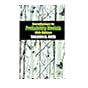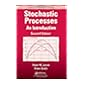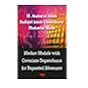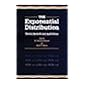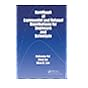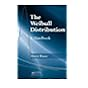519.2 ROI 2010 Introduction to probability models / 519.2 ROI 2010 Introduction to probability models / 519.23 JOS 2010 Stochastic processes : 519.233 ISM 2009 Markov models with covariate dependence for repeated measures / 519.24 EXP 1995 The exponential distribution : 519.24 PAH 2006 Handbook of exponential and related distributions for engineers and scientists / 519.24 RIW 2009 The Weibull distribution :

TOC Repeated measures data -- Markov chain : some preliminaries -- Generalized linear models and logistic regression -- Covariate dependent two state first order Markov model -- Covariate dependent two state second order Markov model -- Covariate dependent two state higher order Markov model -- Multistate first order Markov model with covariate dependence -- Multistate Markov model of higher order with covariate dependence -- An alternative formulation based on Chapman-Kolmogorov equation -- Additional inference procedures -- Generalized linear model formulation of higher order Markov models -- Marginal and conditional models.

AS

Sagar Shahanawaz

There are no comments on this title.